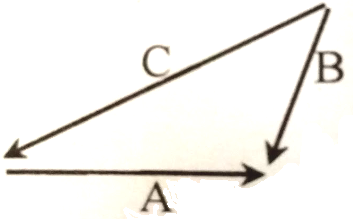If the angle between the unit vectors $\stackrel{^}{\mathrm{a}}$ and $\stackrel{^}{\mathrm{b}}$ is $60°$, then $|\stackrel{^}{\mathrm{a}}-\stackrel{^}{\mathrm{b}}|$ is :

(A)  0

(B)  1

(C)  2

(D)  4

Concept Questions :-

Resultant of Vectors
High Yielding Test Series + Question Bank - NEET 2020

Difficulty Level:

For the figure -1.  A+B=C

2.  B+C=A

3.  C+A=B

4.  A+B+C=0

Concept Questions :-

Resultant of Vectors
High Yielding Test Series + Question Bank - NEET 2020

Difficulty Level:

Two constant forces  and  act on a body and displace it from the position  to the position . What is the work done?

(A)  9 Joule

(B)  41 Joule

(C)  -3 Joule

(D)  None of these

Concept Questions :-

Scalar Product
High Yielding Test Series + Question Bank - NEET 2020

Difficulty Level:

The two vectors $\mathrm{A}=2\stackrel{^}{\mathrm{i}}+\stackrel{^}{\mathrm{j}}+3\stackrel{^}{\mathrm{k}}$ and $\mathrm{B}=7\stackrel{^}{\mathrm{i}}-5\stackrel{^}{\mathrm{j}}-3\stackrel{^}{\mathrm{k}}$ are -

1.  parallel

2.  perpendicular

3.  anti-parallel

4.  None of these

Difficulty Level:

Two vectors $\mathrm{P}=2\stackrel{^}{\mathrm{i}}+\mathrm{b}\stackrel{^}{\mathrm{j}}+2\stackrel{^}{\mathrm{k}}$ and $\mathrm{Q}=\stackrel{^}{\mathrm{i}}+\stackrel{^}{\mathrm{j}}+\stackrel{^}{\mathrm{k}}$ will be perpendicular if -

(A)  b=0

(B)  b=1

(C)  b=2

(D)  b=-4

Concept Questions :-

Scalar Product
High Yielding Test Series + Question Bank - NEET 2020

Difficulty Level:

A vector perpendicular to $\left(4\stackrel{^}{\mathrm{i}}-3\stackrel{^}{\mathrm{j}}\right)$ is -

(A)  $4\stackrel{^}{\mathrm{i}}+3\stackrel{^}{\mathrm{j}}$

(B)  $7\stackrel{^}{\mathrm{k}}$

(C)  $6\stackrel{^}{\mathrm{i}}$

(D)  $3\stackrel{^}{\mathrm{i}}-4\stackrel{^}{\mathrm{j}}$

Concept Questions :-

Scalar Product
High Yielding Test Series + Question Bank - NEET 2020

Difficulty Level:

Angle that the vector $\mathrm{A}=2\stackrel{^}{\mathrm{i}}+3\stackrel{^}{\mathrm{j}}$ makes with y-axis is -

(A)

(B)

(C)

(D)

Concept Questions :-

Scalar Product
High Yielding Test Series + Question Bank - NEET 2020

Difficulty Level:

A vector A points, vertically upward and, B points towards north. The vector product $\mathrm{A}×\mathrm{B}$ is -

1.  along west

2.  along east

3.  zero

4.  vertically downward

Concept Questions :-

Vector Product
High Yielding Test Series + Question Bank - NEET 2020

Difficulty Level:

The linear velocity of a rotating body is given by $\mathrm{v}=\mathrm{\omega }×\mathrm{r}$, where $\mathrm{\omega }$ is the angular velocity and r is the radius vector. The angular velocity of a body $\mathrm{\omega }=\stackrel{^}{\mathrm{i}}-2\stackrel{^}{\mathrm{j}}+2\stackrel{^}{\mathrm{k}}$ and their radius vector  is -

(A)

(B)

(C)

(D)

Concept Questions :-

Vector Product
High Yielding Test Series + Question Bank - NEET 2020

Difficulty Level:

The displacement x of a particle along a straight line at time t is given by $\mathrm{x}={\mathrm{a}}_{0}+{\mathrm{a}}_{1}\mathrm{t}+{\mathrm{a}}_{2}{\mathrm{t}}^{2}$. The acceleration of the particle is

(A)  ${\mathrm{a}}_{0}$

(B)  ${\mathrm{a}}_{1}$

(C)  $2{\mathrm{a}}_{2}$

(D)  ${\mathrm{a}}_{2}$

Concept Questions :-

Differentiation